﻿ 一种水下航行器运动自导航及轨迹跟踪方法
 舰船科学技术2019, Vol. 41Issue (4): 94-99PDF

A method of moving self-navigation and tracking for auv
GAO Qing-ze
The 760 Research Institute of CSIC, Dalian 116013, China
Abstract: Underwater track is one of the important parameters of underwater moving object. We can calculate the speed and radius of gyration by the track.This paper introduces a method of self-navigation and tracking for AUV and the engineering realization, which adopts the principle of acoustic positioning. It is possible for AUV and shipborne platform to grasp the underwater position information accurately. It is of great significance for the AUV to realize self-navigation and position correction and for the shore station command personnel to grasp the position,speed and maneuverability of the AUV in real time.
Key words: AUV     acoustic positioning     track tracking     engineering realization
0 引　言

AUV是水下机器人的一种，是无缆式水下机器人，习惯上称为自主式水下航行器（Autonomous Underwater Vehicle，AUV），近年来AUV作为一种别具特色的海洋应用装备在海洋物探、定位打捞、军事侦察等领域呈现出强劲的发展势头，正成为一种新的隐蔽性好、机动性高的水下作业平台，已经具有相当的搭载能力和水下续航能力，但由于海水对电磁波（包括可见光在内）的吸收和反射等效应，AUV等水下航行器要实现精确的定位和导航是一件很高难度的事情。目前水下自主航行器的水下自身定位导航都是以惯性导航为基础，主要依靠陀螺仪系统，精确地感知水下自主航行器运动姿态的变化，结合加速度传感器，通过实时速度对时间的积分推算水下自主航行器在水下行进的距离和方向从而解算出自身的位置。这种技术的局限性在于测量误差一定存在，并且随着时间积分在不断累积和放大。

1 轨迹跟踪方法介绍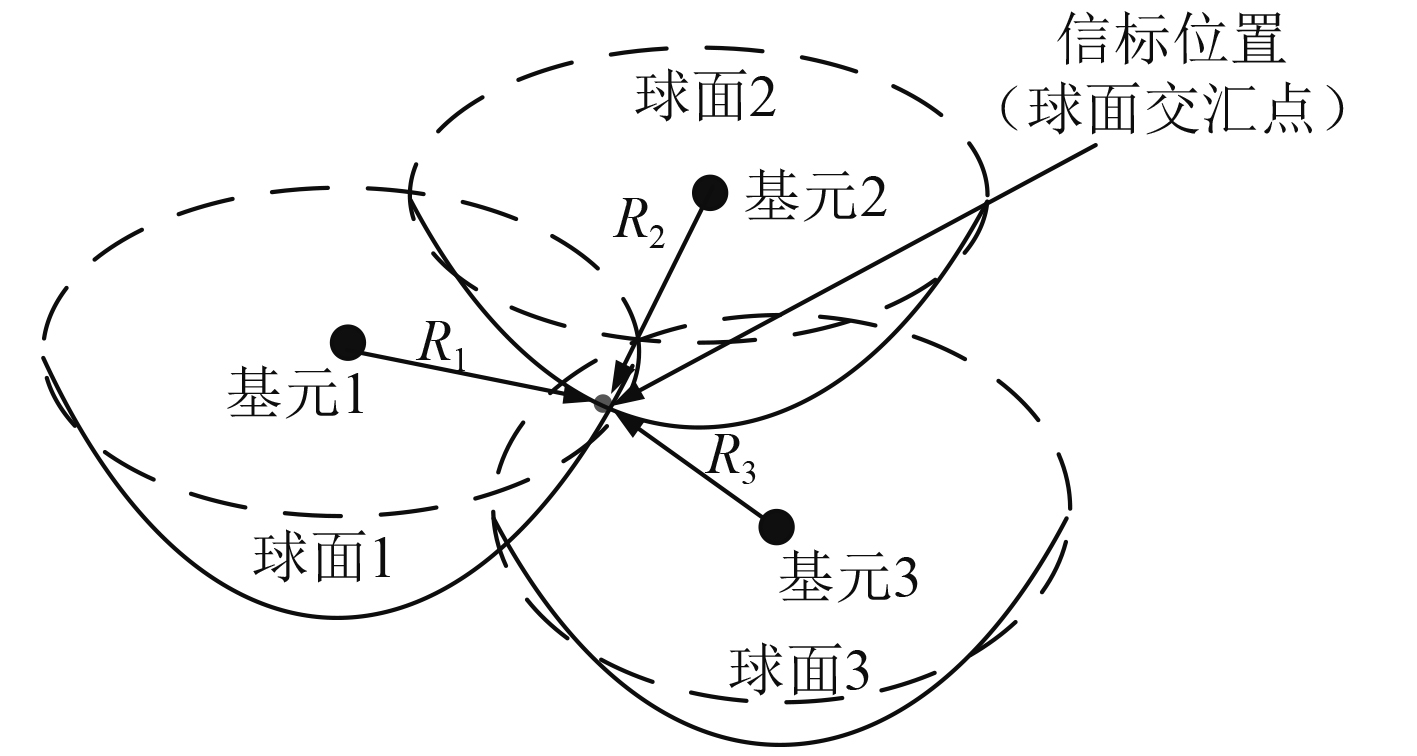图 1 长基线三圆定位原理数学模型 Fig. 1 Mathematical model of long baseline three-circle position principle
 ${R_i} = c \cdot \Delta {t_i} = \sqrt {{{(x - {X_i})}^2} + {{(y - {Y_i})}^2} + {{(z - {Z_i})}^2}}\text{。}$ (1)

2 定位精度分析 2.1 固定目标定位精度分析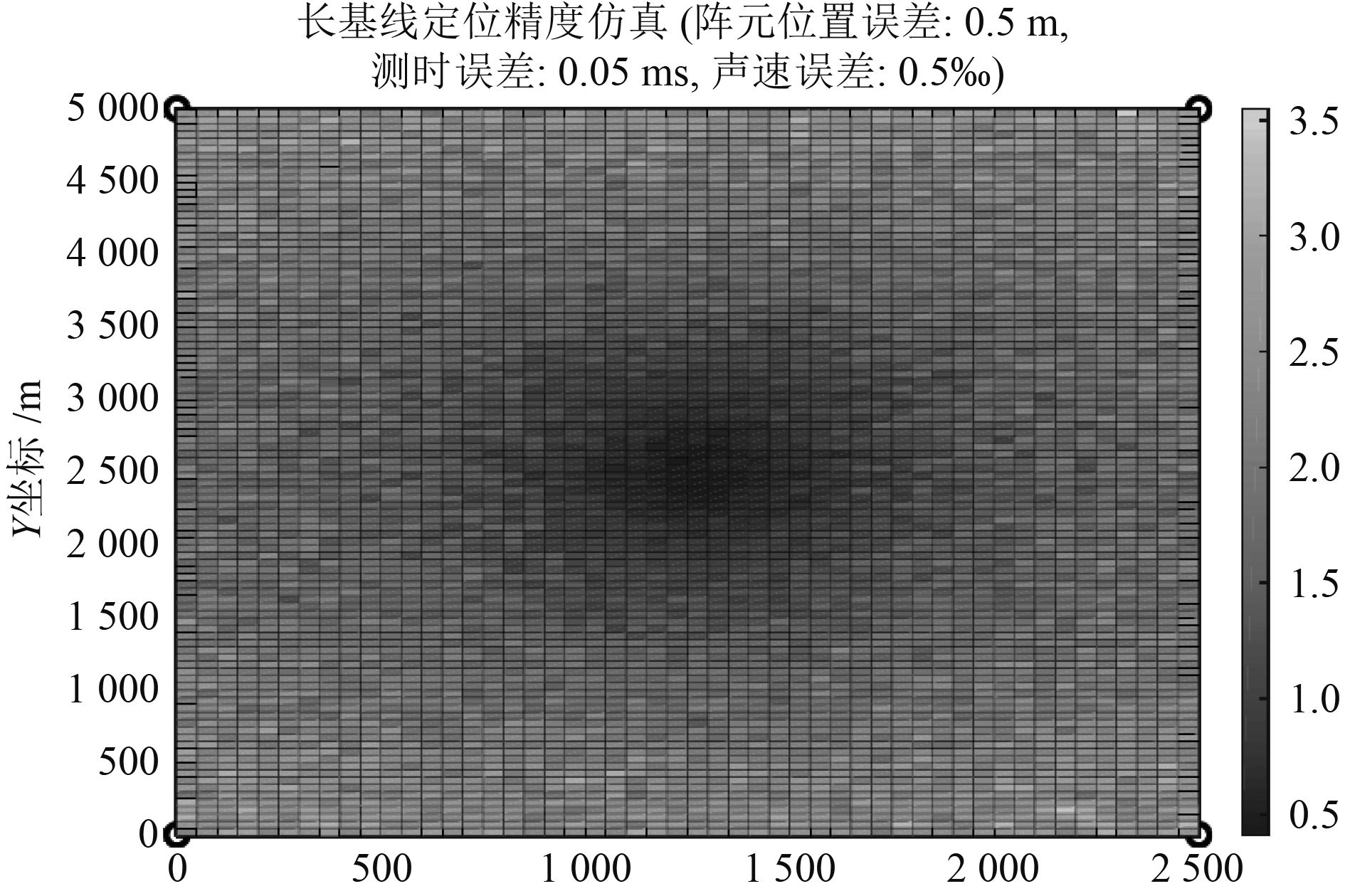图 2 固定目标精度仿真结果 Fig. 2 Simulation results of fixed target precision

2.2 运动目标定位精度分析

 $\Delta {t_2} = \frac{{{V_2}({L_2} - {L_1})}}{{1\;500}}/1\;500\text{。}$ (2)

 $\Delta {t_3} = \frac{{{V_3}({L_3} - {L_1})}}{{1\;500}}/1\;500\text{。}$ (3)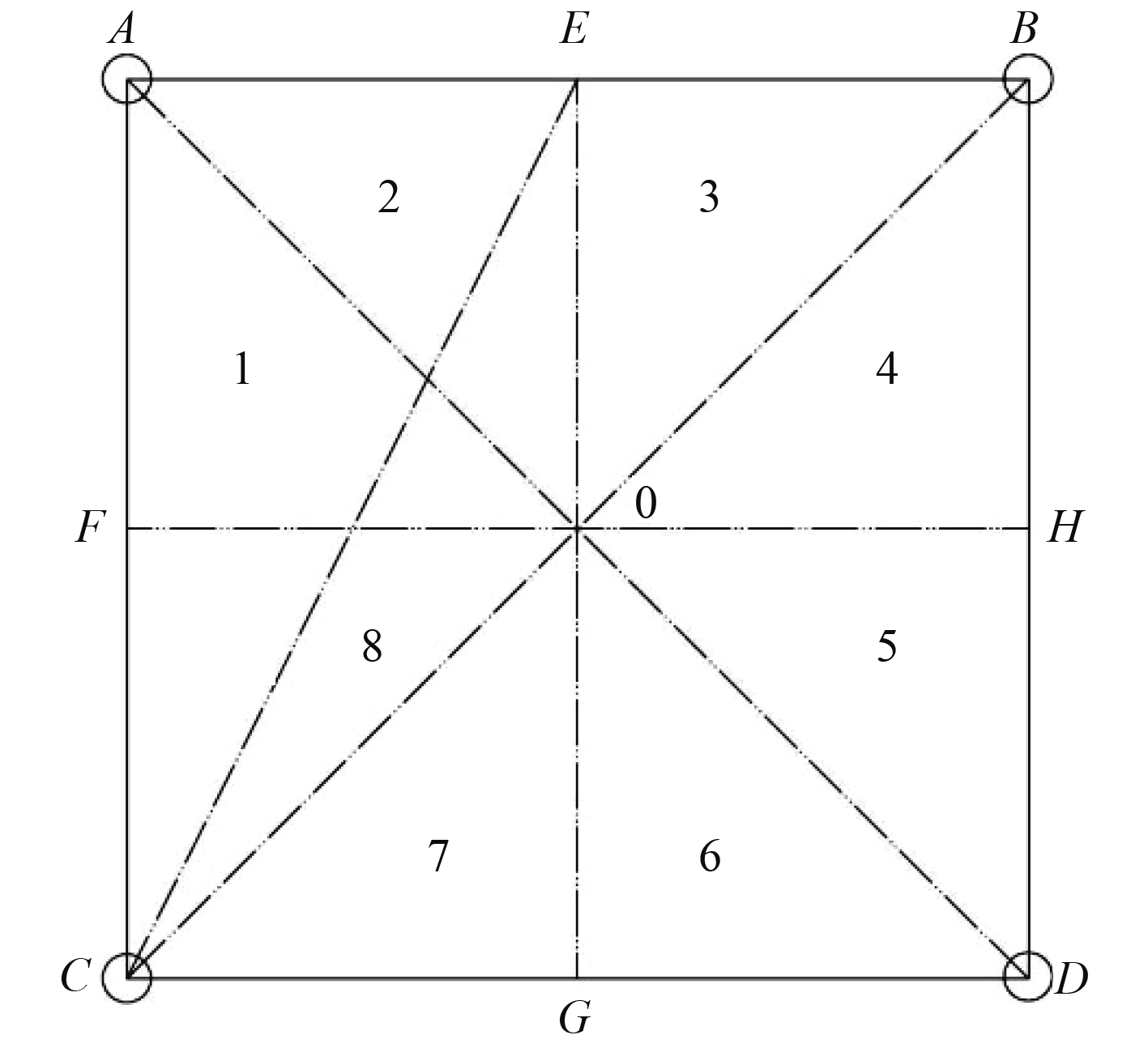图 3 正方形阵 Fig. 3 Square array

 $\Delta {t_D} = \frac{{{V_D}(\sqrt 2 - 1)L}}{{1\;500}}/1\;500\text{，}$ (4)

 $\Delta {t_D} = \frac{{{V_D}(\sqrt 5 /2 - 1/2)L}}{{1\;500}}/1\;500\text{，}$ (5)

 $\Delta {t_D} = \frac{{{V_D}(\sqrt 2 - 1)L}}{{1\;500}}/1\;500\text{。}$ (6)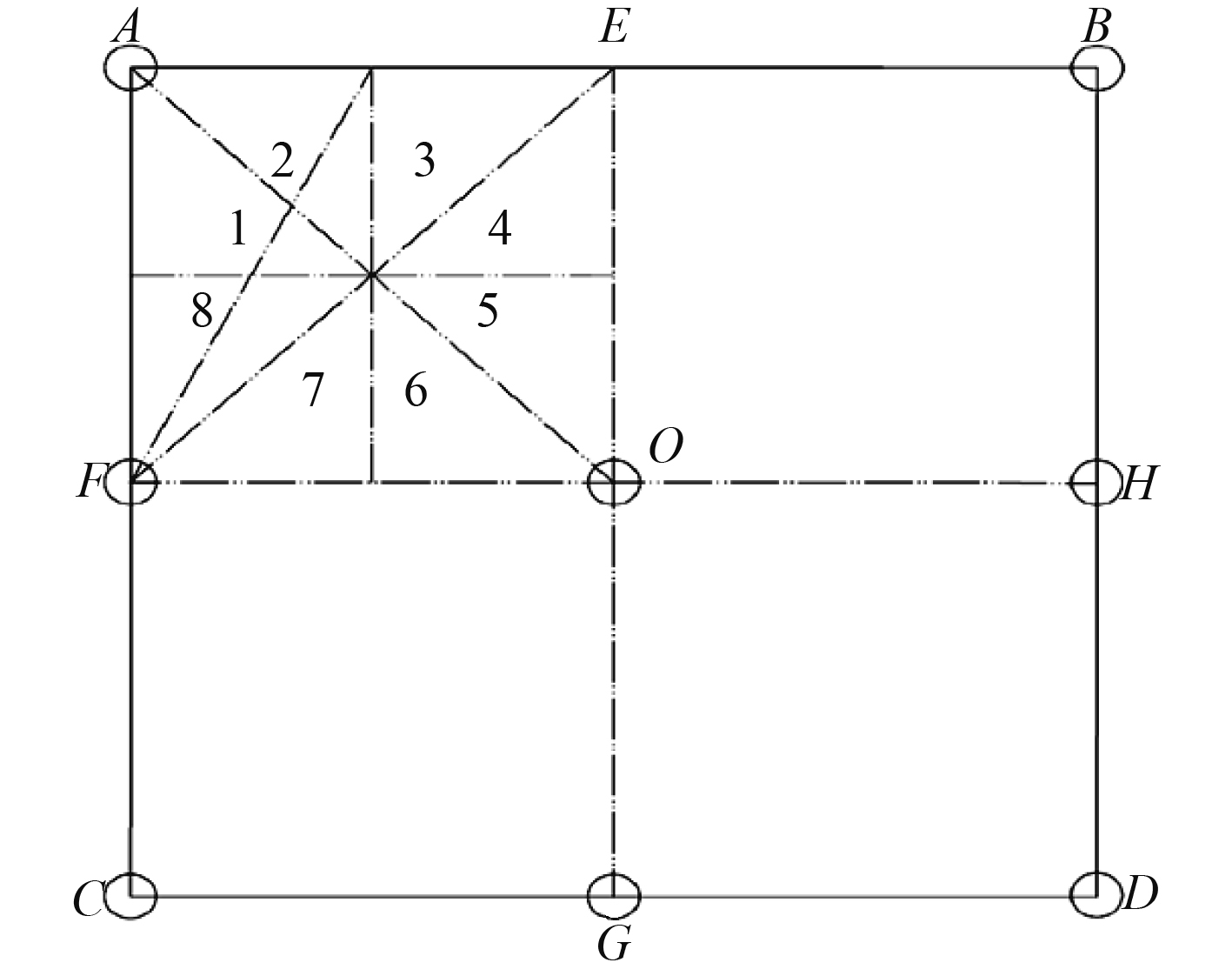图 4 正方形9阵元 Fig. 4 Square 9-element array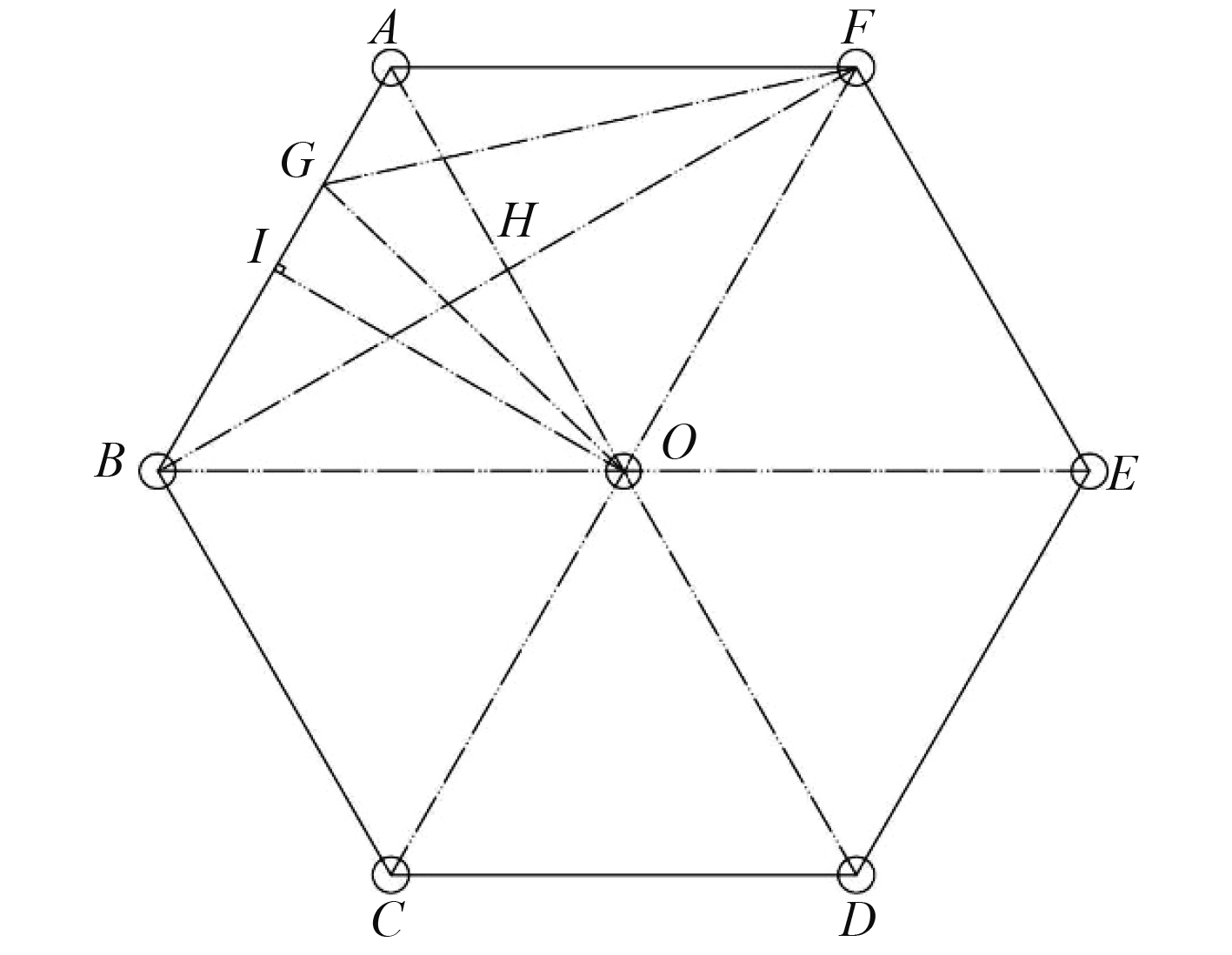图 5 正六边形阵 Fig. 5 Normal hexagonal array

 $\Delta {t_o} = \frac{{{V_o}(\sqrt 3 /2 - 1/2)L}}{{1\;500}}/1\;500\text{，}$ (7)

 $\left( {{{GO - GA}}} \right){{ = }}\left( {{{GF - GB}}} \right)\text{，}$

GO-GA）为在A B O三阵元定位时，最长距离减去最短距离；（GF-GB）为在B O F三阵元定位时，最长距离减去最短距离。

 $\Delta {t_o} = \frac{{{V_o} \cdot 0.543 \cdot L}}{{1\;500}}/1\;500\text{，}$ (8)

G的位置满足AG=0.338 L。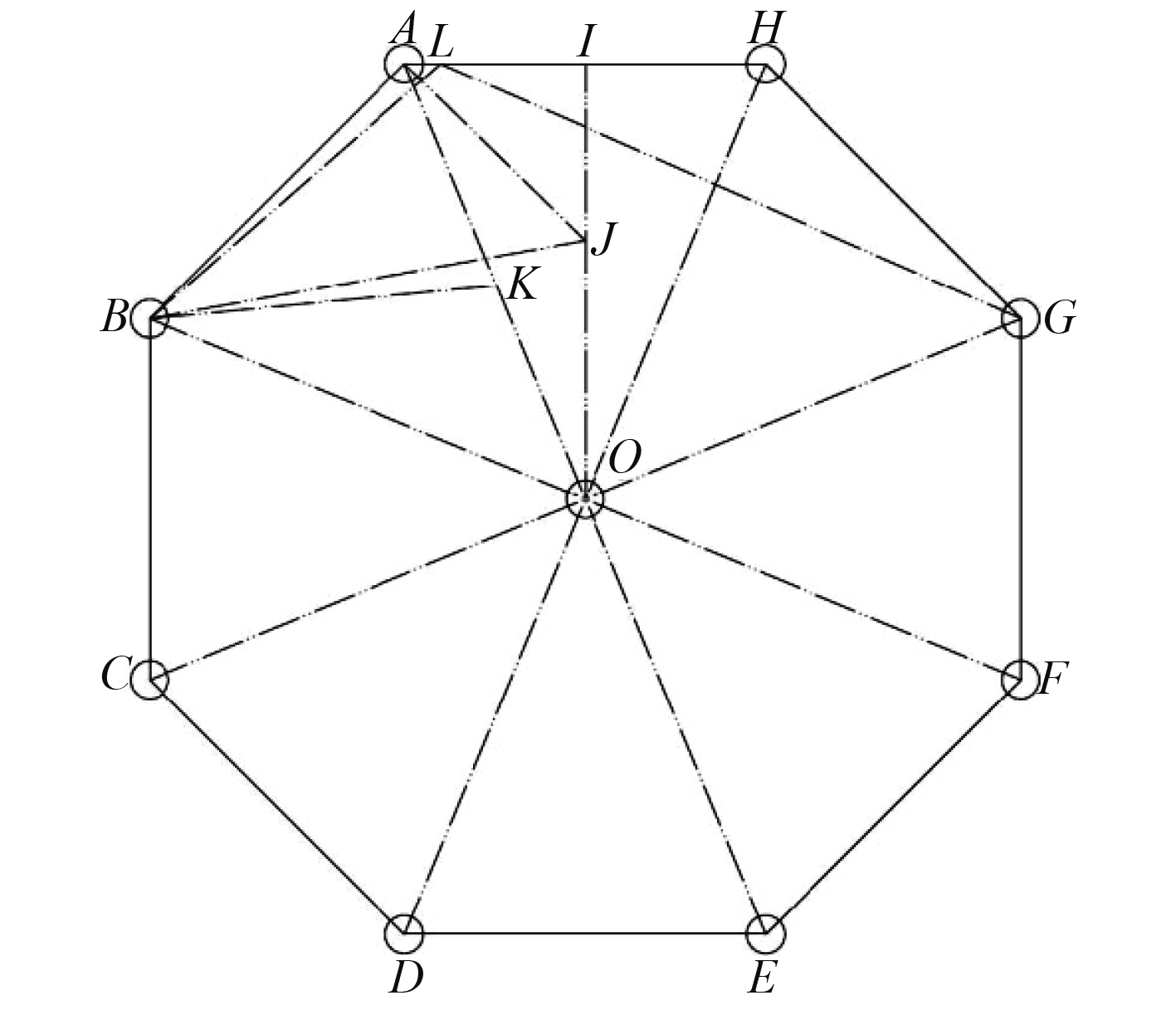图 6 正八边形阵 Fig. 6 Normal octagonal array

 $\Delta {t_o} = \frac{{{V_o} \cdot (0.937 - 0.541) \cdot L}}{{1\;500}}/1\;500\text{，}$ (9)

 $\Delta {t_o} = \frac{{{V_o} \cdot 0.543 \cdot L}}{{1\;500}}/1\;500\text{。}$ (10)

 $\Delta {t_o} = \frac{{{V_o} \cdot (0.96 - 0.512) \cdot L}}{{1\;500}}/1\;500\text{，}$ (11)

 $\Delta {t_o} = \frac{{{V_o} \cdot 0.448 \cdot L}}{{1\;500}}/1\;500\text{。}$ (12)

 $\Delta {t_o} = \frac{{{V_o} \cdot (0.737 - 0.5) \cdot L}}{{1\;500}}/1\;500\text{，}$ (13)

 $\Delta {t_o} = \frac{{{V_o} \cdot 0.237 \cdot L}}{{1\;500}}/1\;500\text{。}$ (14)

 $\Delta {t_o} = \frac{{{V_o} \cdot 0.448 \cdot L}}{{1\;500}}/1\;500{\text{。}}$

 $\Delta {t_D} = \frac{{{V_D}(\sqrt 5 /2 - 1/2)L}}{{1\;500}}/1\;500 = \frac{{{V_D} \cdot 0.618 \cdot L}}{{1\;500}}/1\;500\text{，}$ (15)

 $\Delta {t_o} = \frac{{{V_o} \cdot 0.543 \cdot L}}{{1\;500}}/1\;500\text{，}$ (16)

 $\Delta {t_o} = \frac{{{V_o} \cdot 0.448 \cdot L}}{{1\;500}}/1\;500\text{。}$ (17)

3 工程实现方法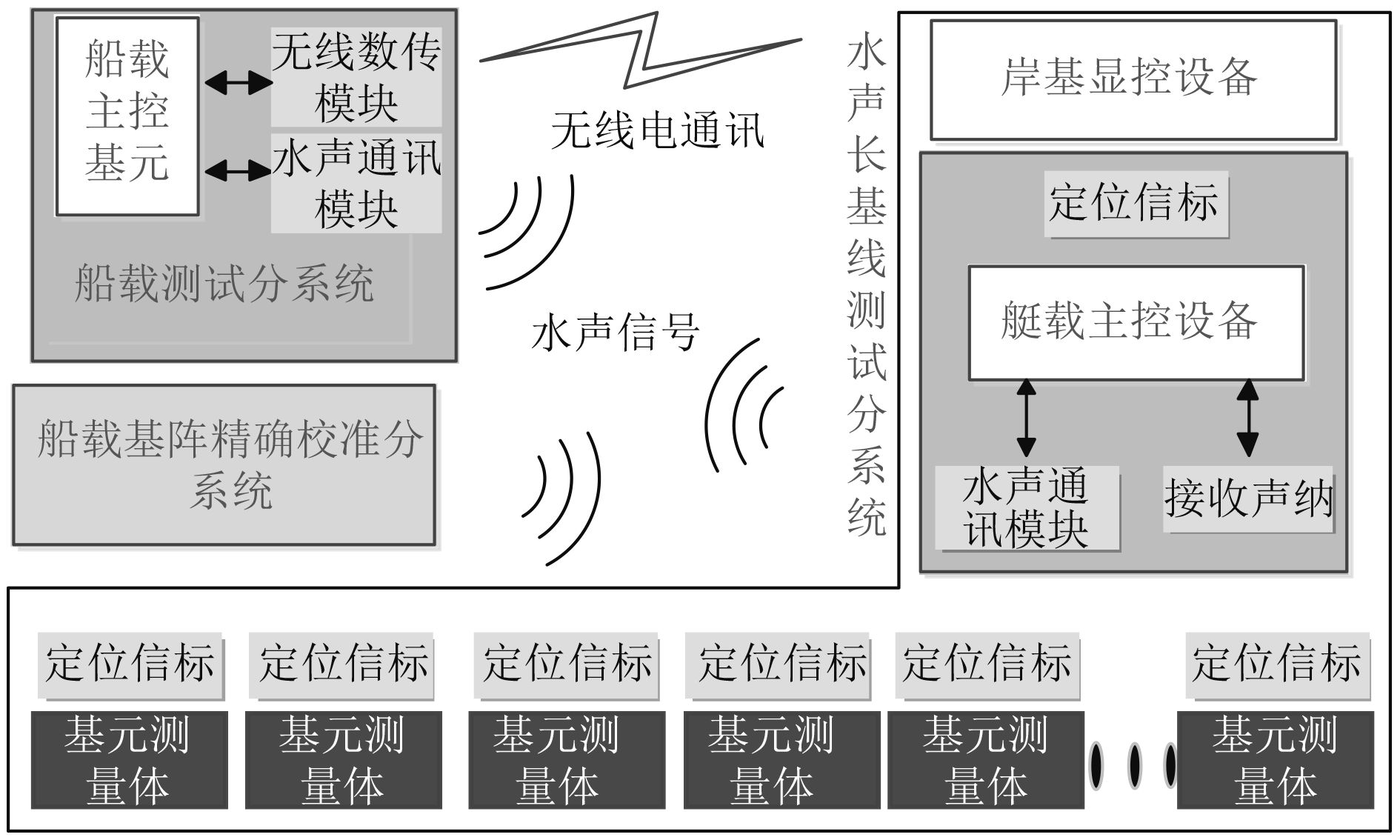图 7 系统组成框图 Fig. 7 System composition block diagram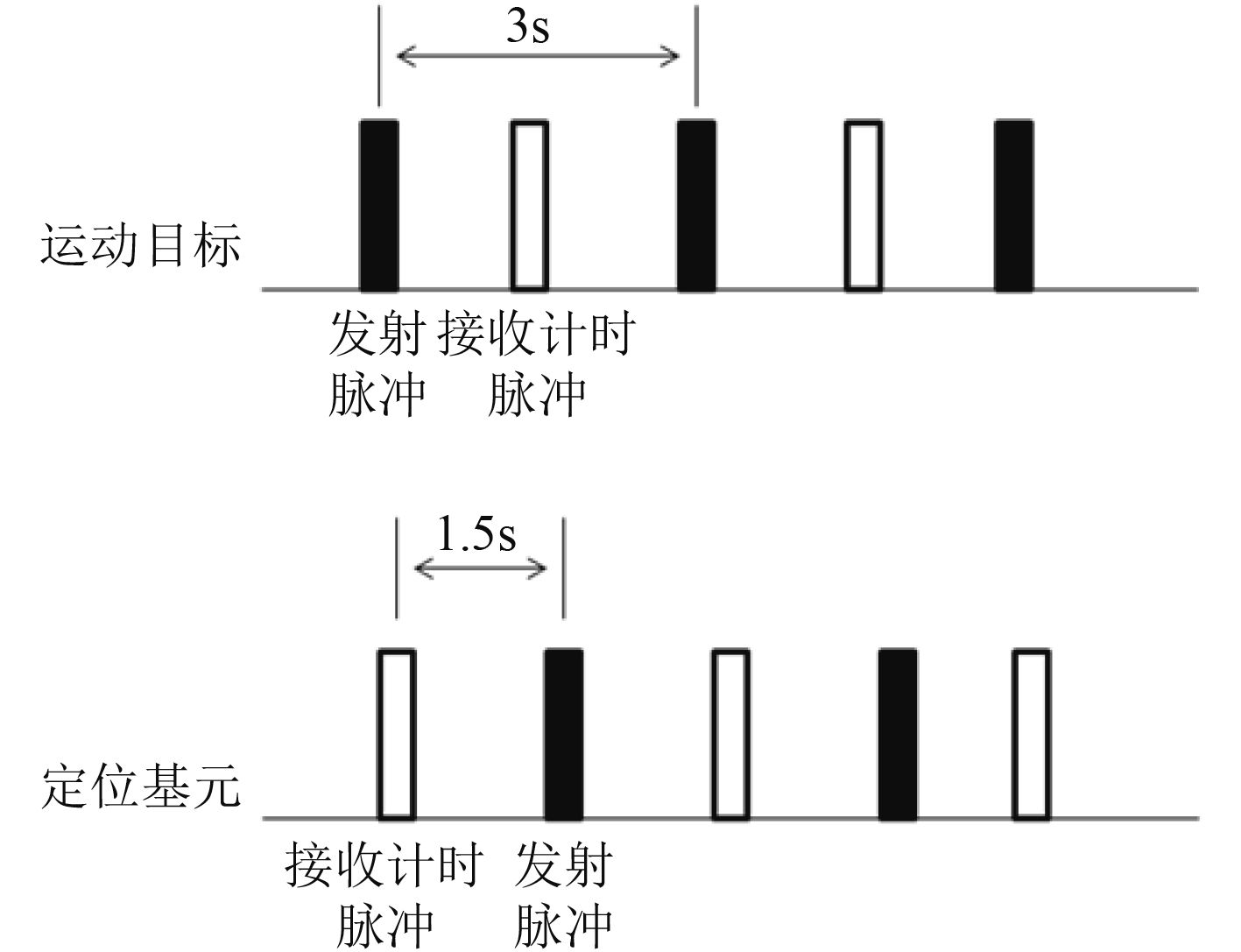图 8 系统工作时序图 Fig. 8 System working sequence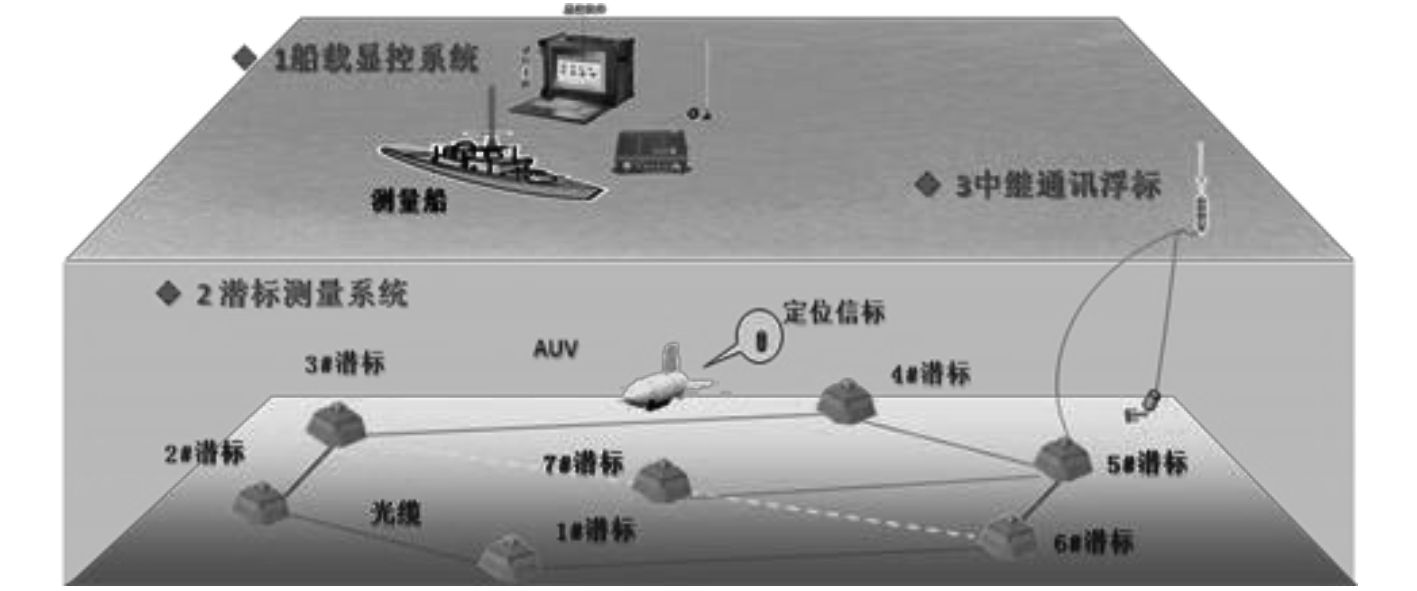图 9 系统工作态势图 Fig. 9 System working situation map
4 结　语

  马伟锋, 胡震. AUV的研究现状与发展趋势[J]. 火力与指挥控制, 2008, 33(6): 10-13. DOI:10.3969/j.issn.1002-0640.2008.06.003  江南, 黄建国, 李姗. 长基线水下目标定位新技术研究[J]. 仪器仪表学报, 2004, 25(4): 77-80.  于运治, 姜璐, 郭志强. 一种水下精确定位方法及其误差分析[J]. 四川兵工学报, 2011, 32(9): 121-123. DOI:10.3969/j.issn.1006-0707.2011.09.039  蔡惠智, 刘云涛, 蔡慧, 等. 水声通信及其研究进展[J]. 物理, 2006, 35(12): 1038-1042. DOI:10.3321/j.issn:0379-4148.2006.12.011  许肖梅. 水声通讯与水声网络的发展与应用[J]. 声学技术, 2009, 28(6): 811-814. DOI:10.3969/j.issn1000-3630.2009.06.026  陈云飞, 李桂娟, 贾兵, 等. 水下合作目标三维定位技术[J]. 舰船科学技术, 2010, 32(5): 48-51.  吴永亭, 周兴华, 杨龙. 水下声学定位系统及其应用[J]. 海洋测绘, 2003, 23(4): 18-21. DOI:10.3969/j.issn.1671-3044.2003.04.006  陈逸伦. 一种基于GPS实现水下定位的有效方法[J]. 科学技术与工程, 2011, 11(31): 7754-7757. DOI:10.3969/j.issn.1671-1815.2011.31.035